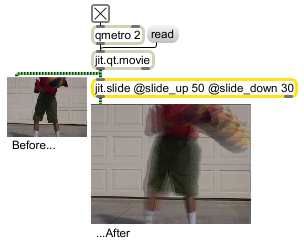jit.slide

Cellwise temporal envelope follower

Description

The jit.scanslide object performs cellwise temporal envelope following using the formula y (n) = y (n-1) + ((x (n) - y (n-1))/slide).

Matrix Operator

matrix inputs:1, matrix outputs:1
 Name IOProc Planelink Typelink Dimlink Plane Dim Type out n/a 1 1 1 1 1 char long float32 float64

Attributes

Name Type g/s Description
slide_down float The slide down factor (default = 1.) Note that down refers not to spatial change along the y axis, but rather with respect to amplitude (e.g. brightness of color channel for image data).
slide_up float The slide up factor (default = 1.) Note that up refers not to spatial change along the y axis, but rather with respect to amplitude (e.g. brightness of color channel for image data).

Examples